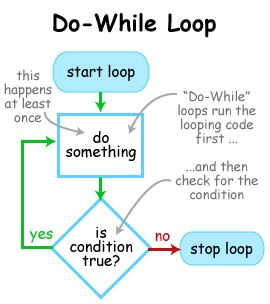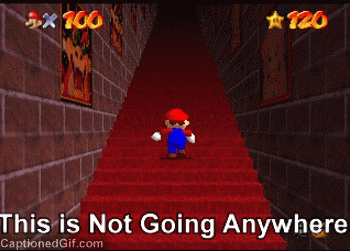# Java Do While Loop With Examples – Java Tutoring

Do while in Java Everything you need to know about Java do while with a flowchart and example program with output and complete methods and basics. – Learn more Java Tutorials and Beginners Programs.

So Basically what are loops In Java?

• Def : ‘Executing a set of statements‘ process is known as looping. We have three types of looping constructs in Java. These looping statements are also called iterative statements. Check out those three Looping statements in Java – Followed Explanation part about java do while with examples and sample program with output.1. while loop
2. for loop
3. do while loop

As you might know that all the three are used primarily for the same purpose and the difference is in their syntax , the basic difference is :

• Because of the syntactical differences, their behavior may differ a little bit. We will see the differences soon about do while, for , while.

More than the behavior, it is programmer’s choice about what looping constructor to use when.

## Do While In Java Basics – Everything You Need To Know With Examples

Basic Definition :

• Do-while is similar to while loop but, the condition is checked at the end of each iteration (in while-loop, the condition is checked at the beginning of each iteration) Understand? Hope you do  😛

Basic Flow Chart Of Do while loop in java• Flowchart : In a while, if the condition is false for the first time the loop body is not at all executed. But in do-while the loop body is executed at least once even though the condition is false for the first time – Check the complete list of differences between do-while and while with examples.

Syntax For Do-while Basics :

• When control comes into this loop, with any condition checking, it enters into loop body and executes it. Then it comes to condition part check and evaluates.

If it results in true, the control goes back to loop body and executes it. Then it comes to condition part. This process of ‘executing body’ and ‘condition checking’ is repeated as long as the condition results in true. When the condition results in false, the control comes out of the loop and executes the statement immediately following the loop. For more information about Java do while for the above syntax how it works do check it out the following example with sample output program:

So you got an idea about the java do while basics , here we share 7 unique examples with outputs do check it out.

Example Program – 1 ( Executing without checking condition for the first time )

Output:

Example Part – 2 (  Executing with checking state )

An explanation for the above examples for java do while :

In the above sample example, value of a is 1 by the time the control comes into the do while  loop. Without any checking, the control enters the loop body and prints 1. Then it is incremented by 1. From there it goes to condition part and evaluates it. As it results in true (1<=3), the control goes back to loop body and prints 2 and then makes a value as 3.

When the next time condition checks, it again results in right (2<=3). So control goes to the body to print 3 and to update a to 4. This time the condition will result in false (4<=3) so the control comes out of the loop, that’s just a basic example here we shared in depth tutorial about the  ” do-while java with examples in different methods .”

Simple Do-while Java Program With OutPut: [ Example – 3 ]

Infinite Loop  😛Output:

IMP : We can have any number of statements in a do-while loop, and all those statements should be enclosed within a set of curly braces.

For example code this how syntax will be in format :

Do while loop Example Program WithOut Infinite Loop – [ Example Program – 4 ]

Output:

Note: The curly braces are optional when we have only one statement as part of the body. So the following examples will mean same.

Example Synatx : 1

Example Syntax :  2 [ Both are same in case if we have only one statement ]

• Generally, a construct/statement ends with a semicolon or a closing curly brace but not with a closing parenthesis. So the following statement is like:
1. if(a<=b)                is not complete
2. if(a<=b);               is complete
3. if(a<=b)}              is not valid as there is no corresponding opening curly brace.

this is why a do-while construct is ended with a semi-colon, and it’s must required.

Java do-while loop statement Example – 7

### Nesting Of Do-While Construction – with Examples

Definition :

• We can have another do-while in a do-while construct:  Nesting of do-while construction. There is no limit to the depth of nesting. A do-while can have any other construct (if, switch, while, for, etc.) inside it – For example, check out the following syntax.

Syntax : Nesting

A [ simple example program – 1 ] with output how nesting in a do while will work under nesting process :

Output:

Another [ Simple Example – 2 ] With Output:

Output:

• We can create an infinite loop by placing a condition that results in true always.

Eg1:   Syntax

Eg2:   Syntax

• We can create variables inside a do-while, but they can be used only within that loop. Those variables were automatically destroyed when the loop ends. So they cannot be used outside the loop.
• We can come out of a loop somewhere from a middle of the body; we can use a break. Similarly, continue can be used to skip statements in the current round (iteration) and go to the next round (iteration).

#### Important Points Do’s and Don’ts  :

1. Minimum one statement is required as part of the body

• do while(m==n);              is not valid
• do ; while(m==n);            is valid
• do System.out.println(“be happy always”); while(m==n);            is valid
• do { } while(m==n);         is valid

2. The condition should always result in a boolean value.

• do { } while(4==8);           is valid
• do { } while(4&&8);         is not valid
• do { } while(true && true);          is valid
• do { } while(1);                  is not valid

3. Condition is required, we should not omit that part.

• do { } while();    is not valid
• do { } while(a<=b);          is valid

A ” do ” should be associated with a corresponding while part. Otherwise, the compiler will raise an error.

Eg:

• do           {              }                                              is not valid
• do           {              } while(condition);         is valid

x

## What is Recursion In Java Programming – JavaTutoring

What is Recursion In Java programming – Here we cover in-depth article to know more ...